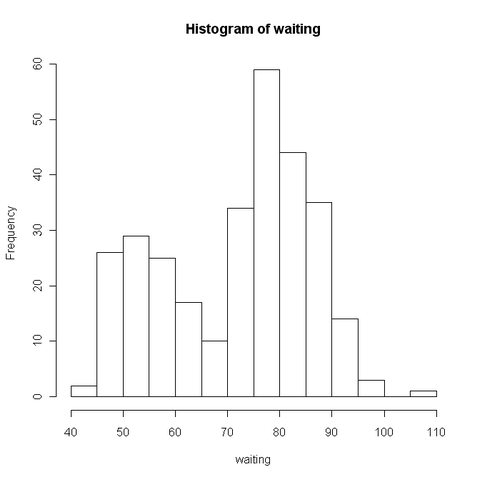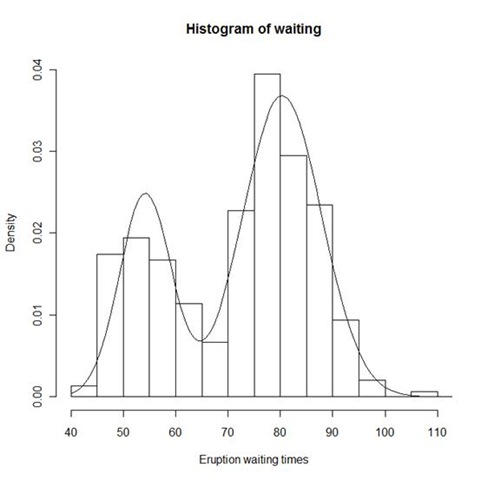# 1. 数据与模型

geyser
waiting  duration
1        80 4.0166667
2        71 2.1500000
3        57 4.0000000
4        80 4.0000000
5        75 4.0000000
......


attach(geyser)


# 2. 模型

hist(waiting)$$f(x)=pN(x_i;\mu_1,\sigma_1)+(1-p)N(x_i;\mu_2,\sigma_2)$$

$$l=\sum_{i=1}^n\log \{pN(x_i;\mu_1,\sigma_1)+(1-p)N(x_i;\mu_2,\sigma_2)\}$$

# 3. 估计

## 3.1. 在R中定义对数似然函数：

# 定义log-likelihood函数
LL <- function(params, data) {
# 参数 params 是一个向量，依次包含了五个参数：p, mu1, sigma1, mu2, sigma2.
# 参数 data 是观测数据
t1 <- dnorm(data, params, params)
t2 <- dnorm(data, params, params)
# 这里的 dnorm() 函数是用来生成正态密度函数的
f <- params * t1 + (1 - params) * t2
# 混合密度函数
ll <- sum(log(f))
# log-likelihood函数
return(-ll)
# nlminb() 函数是最小化一个函数的值，但我们是要最大化log-likeilhood函数，所以需要在 ll 前加个负号
}


## 3.2. 参数估计

# 用 hist 函数找出初始值
hist(waiting, freq = F)
lines(density(waiting))
# 拟合函数####optim####
geyser.res <- nlminb(c(0.5, 50, 10, 80, 10), LL,
data = waiting,
lower = c(0.0001, -Inf, 0.0001, -Inf, -Inf, 0.0001),
upper = c(0.9999, Inf, Inf, Inf, Inf)
)
# 初始值为 p=0.5, mu1=50, sigma1=10, mu2=80, sigma2=10
# LL是被最小化的函数
# data是拟合用的数据
# lower和upper分别指定参数的上界和下界


## 3.3. 估计结果

# 查看拟合的参数
geyser.res$par #  0.3075937 54.2026518 4.9520026 80.3603085 7.5076330 # 拟合的效果 X <- seq(40, 120, length = 100) # 读出估计的参数 p <- geyser.res$par
mu1 <- geyser.res$par sig1 <- geyser.res$par
mu2 <- geyser.res$par sig2 <- geyser.res$par
# 将估计的参数函数代入原密度函数。
f <- p * dnorm(X, mu1, sig1) + (1 - p) * dnorm(X, mu2, sig2)
# 作出数据的直方图
hist(waiting,
probability = T, col = 0, ylab = "Density",
ylim = c(0, 0.04), xlab = "Eruption waiting times"
)
# 画出拟合的曲线
lines(X, f)detach()


# 参考文献：

• l Brian S. Everitt(2002). A Handbook of Statistical Analyses Using S-Plus (Second Edition). CRC Press LLC